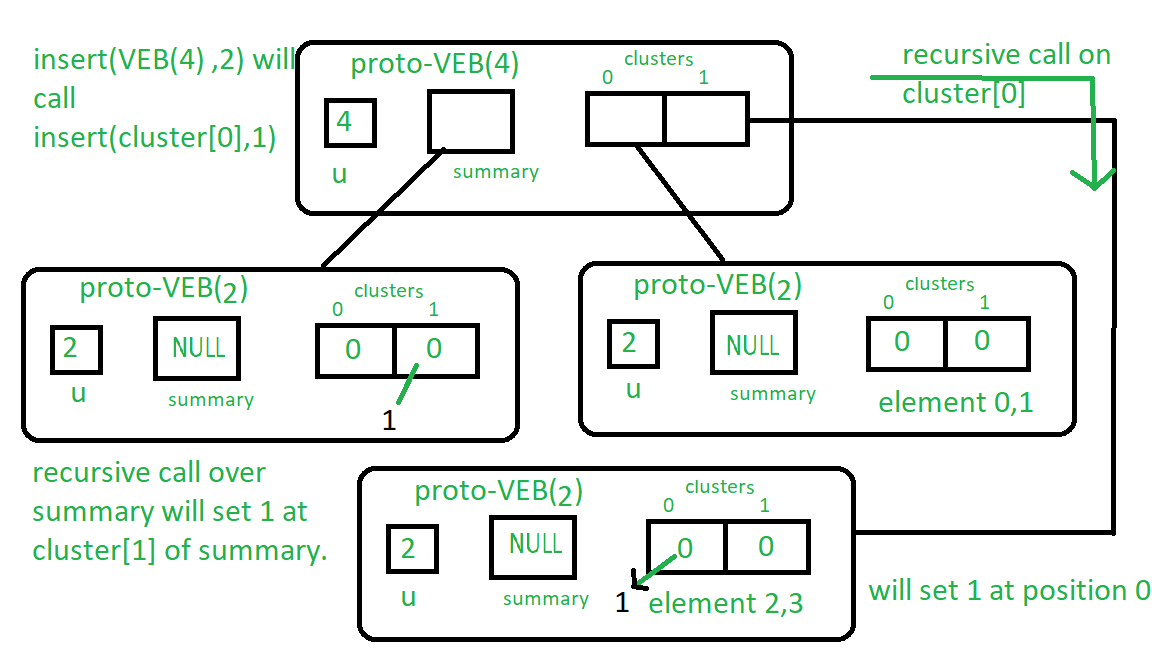# Proto Van Emde Boas Tree | Set 3 | Insertion and isMember Query

• Last Updated : 10 Sep, 2021

Please see previous articles on Proto Van Emde Boas Tree to understand these properly.

Procedure for Insert:

Attention reader! Don’t stop learning now. Get hold of all the important DSA concepts with the DSA Self Paced Course at a student-friendly price and become industry ready.  To complete your preparation from learning a language to DS Algo and many more,  please refer Complete Interview Preparation Course.

In case you wish to attend live classes with experts, please refer DSA Live Classes for Working Professionals and Competitive Programming Live for Students.

1. Base Case: If the size of Proto-VEB is 2 then assign true to the bit array( Here we in code we assign Proto-VEB(1) due to recursive structure and so now it is not nullptr and it act as true ) at the position of key.
2. Until we reach at the base case, we will recursively call insert on cluster containing key and also now we use the key as the position of the key in that cluster instead of the query key.

Example: Let’s insert 2 into Proto-VEB (u=4): From the procedure of insert we will start recursion as size of Proto-VEB is greater than 2 so we recursively call insert() on cluster number 2/which is 1 and it’s position 2%which is 0 so recursive call will be insert(cluster, 0).
And cluster is size 2 Proto-VEB, we reached at the base case so it will assign true at( in code Proto-VEB(1) as true ) cluster 0th place.
Likewise, we will do the same procedure over summary.
See the image below for more clarity:
Follow the instructions written near the boxes from top to bottom.isMember procedure: This procedure returns boolean value according to whether the key is present in Proto-VEB or not. It is quite trivial to understand see the image above to get the idea about it.

1. Base Case: If the Proto-VEB size is 2 then check if bit array value at the key position is true or not and return value accordingly. (In code we check whether pointer at the key position is nullptr or not.)
2. Recursion: we do recursive call over cluster containing key until we reach the base case.

Implementation of above algorithm:

## CPP

 // C++ implementation of the approach#include using namespace std; class Proto_Van_Emde_Boas {public:    // Total number of keys    int universe_size;     // Summary    Proto_Van_Emde_Boas* summary;     // Clusters array of Proto-VEB pointers    vector clusters;     int root(int u)    {        return int(sqrt(u));    }     // Function to return cluster numbers    // in which key is present    int high(int x)    {        return x / root(universe_size);    }     // Function to return position of x in cluster    int low(int x)    {        return x % root(universe_size);    }     // Function to return the index from    // cluster number and position    int generate_index(int cluster, int position)    {        return cluster * root(universe_size) + position;    }     // Constructor    Proto_Van_Emde_Boas(int size)    {        universe_size = size;         // Base case        if (size <= 2) {             // Set summary to nullptr as there is no            // more summary for size 2            summary = nullptr;             // Vector of two pointers            // nullptr in starting            clusters = vector(size, nullptr);        }        else {             // Assigning Proto-VEB(sqrt(u)) to summary            summary = new Proto_Van_Emde_Boas(root(size));             // Creating array of Proto-VEB Tree pointers of size sqrt(u)            // first all nullptrs are going to assign            clusters = vector(root(size), nullptr);             // Assigning Proto-VEB(sqrt(u)) to all its clusters            for (int i = 0; i < root(size); i++) {                clusters[i] = new Proto_Van_Emde_Boas(root(size));            }        }    }}; // Function that returns true if the// key is present in the treebool isMember(Proto_Van_Emde_Boas* helper, int key){    // If key is greater then universe_size then    // returns false    if (key >= helper->universe_size)        return false;     // If we reach at base case    // the just return whether    // pointer is nullptr then false    // else return true    if (helper->universe_size == 2) {        return helper->clusters[key];    }    else {         // Recursively go deep into the        // level of Proto-VEB tree using its        // cluster index and its position        return isMember(helper->clusters[helper->high(key)],                        helper->low(key));    }} // Function to insert a key in the treevoid insert(Proto_Van_Emde_Boas*& helper, int key){    // If we reach at base case    // then assign Proto-VEB(1) in place    // of nullptr    if (helper->universe_size == 2) {        helper->clusters[key] = new Proto_Van_Emde_Boas(1);    }    else {         // Recursively using index of cluster and its        // position in cluster        insert(helper->clusters[helper->high(key)],               helper->low(key));         // Also do the same recusion in summary VEB        insert(helper->summary, helper->high(key));    }} // Driver codeint main(){    Proto_Van_Emde_Boas* hello = new Proto_Van_Emde_Boas(4);     cout << isMember(hello, 3);     insert(hello, 3);     cout << isMember(hello, 3);}

Insert Algorithm Complexity Recurrence:

T(u) = 2T() + O(1)

This algorithm runs in O(log2(u)) worst-case time.
isMember Algorithm Complexity Recurrence:

T(u) = T() + O(1)

This algorithm runs in O(log2(log2(u))) worst-case time.

My Personal Notes arrow_drop_up